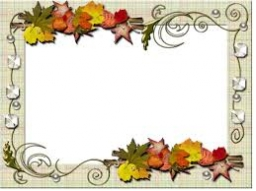# A photograph

A photograph will stick to a white square letter with a x cm length. The photo is 3/4 x cm long and 20 cm wide than the width of the paper. The surface of the remaining paper surrounding the photograph is 990 cm2. Find the size of paper and photo.

Correct result:

a =  39.846 cm
b =  29.885 cm
c =  20 cm

#### Solution:

$S_{1}=a^2 \ \\ b=3/4 \cdot \ a \ \\ c=20 \ \\ S_{2}=b \cdot \ c \ \\ S_{1}-S_{2}=990 \ \\ \ \\ a^2 - 3/4*a*20=990 \ \\ \ \\ a^2 - 3/4 \cdot \ a \cdot \ 20=990 \ \\ a^2 -15a -990=0 \ \\ \ \\ p=1; q=-15; r=-990 \ \\ D=q^2 - 4pr=15^2 - 4\cdot 1 \cdot (-990)=4185 \ \\ D>0 \ \\ \ \\ a_{1,2}=\dfrac{ -q \pm \sqrt{ D } }{ 2p }=\dfrac{ 15 \pm \sqrt{ 4185 } }{ 2 }=\dfrac{ 15 \pm 3 \sqrt{ 465 } }{ 2 } \ \\ a_{1,2}=7.5 \pm 32.3457879793 \ \\ a_{1}=39.8457879793 \ \\ a_{2}=-24.8457879793 \ \\ \ \\ \text{ Factored form of the equation: } \ \\ (a -39.8457879793) (a +24.8457879793)=0 \ \\ \ \\ a=a_{1}=39.8458=39.846 \ \text{cm}$

Checkout calculation with our calculator of quadratic equations.

$b=3/4 \cdot \ a=3/4 \cdot \ 39.8458=29.885 \ \text{cm}$
$c=20 \ \text{cm} \ \\ \ \\ S_{1}=a^2=39.8458^2 \doteq 1587.7037 \ \text{cm}^2 \ \\ S_{2}=b \cdot \ c=29.8845 \cdot \ 20=\dfrac{ 5977 }{ 10 }=597.7 \ \text{cm}^2$Our examples were largely sent or created by pupils and students themselves. Therefore, we would be pleased if you could send us any errors you found, spelling mistakes, or rephasing the example. Thank you!

Leave us a comment of this math problem and its solution (i.e. if it is still somewhat unclear...):Be the first to comment!Tips to related online calculators
Looking for help with calculating roots of a quadratic equation?
Do you have a linear equation or system of equations and looking for its solution? Or do you have quadratic equation?

#### You need to know the following knowledge to solve this word math problem:

We encourage you to watch this tutorial video on this math problem:

## Next similar math problems:

• A bottleA bottle full of cola weighs 1,320 g. If we drink three-tenths of it, it will weigh 1,008g. How much does an empty bottle weigh?
• Save trees25 tons of old paper will save 1,600 trees. How many tons of paper is needed to save the 32 trees in the park?
• Triangle in a squareIn a square ABCD with side a = 6 cm, point E is the center of side AB and point F is the center of side BC. Calculate the size of all angles of the triangle DEF and the lengths of its sides.
• Trip with compassDuring the trip, Peter went 5 km straight north from the cottage, then 12 km west and finally returned straight to the cottage. How many kilometers did Peter cover during the whole trip?
• 1 page1 page is torn from the book. The sum of the page numbers of all the remaining pages is 15,000. What numbers did the pages have on the page that was torn from the book?
• Cube surfce2volumeCalculate the volume of the cube if its surface is 150 cm2.
• Two chordsFrom the point on the circle with a diameter of 8 cm, two identical chords are led, which form an angle of 60°. Calculate the length of these chords.Calculate the content of a regular 15-sides polygon inscribed in a circle with radius r = 4. Express the result to two decimal places.
• Lookout towerHow high is the lookout tower? If each step was 3 cm lower, there would be 60 more of them on the lookout tower. If it was 3 cm higher again, it would be 40 less than it is now.
• Aquarium heightHow high does the water in the aquarium reach, if there are 36 liters of water in it? The length of the aquarium is 60 cm and the width is 4 dm.
• In theIn the rectangle ABCD, the distance of its center from the line AB is 3 cm greater than from the line BC. The circumference of the rectangle is 52 cm. Calculate the contents of the rectangle. Express the result in cm2.
• DodecagonCalculate the size of the smaller of the angles determined by lines A1 A4 and A2 A10 in the regular dodecagon A1A2A3. .. A12. Express the result in degrees.How many times does the surface of a sphere decrease if we reduce its radius twice?An observer standing west of the tower sees its top at an altitude angle of 45 degrees. After moving 50 meters to the south, he sees its top at an altitude angle of 30 degrees. How tall is the tower?The observer sees the base of the tower 96 meters high at a depth of 30 degrees and 10 minutes and the top of the tower at a depth of 20 degrees and 50 minutes. How high is the observer above the horizontal plane on which the tower stands?Calculate the volume of a spherical segment 18 cm high. The diameter of the lower base is 80 cm, the upper base 60 cm.Calculate the volume of a regular hexagonal prism whose body diagonals are 24cm and 25cm long.